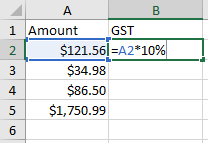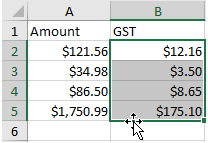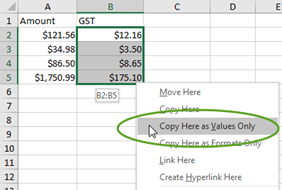Excel tutorial: Pasting Values with Drag and Drop

### Pasting the values from a formula (so that the numbers never change) has always involved several steps. Often you want to calculate an answer, but store the number, not the calculation.

However, with this tip, you can easily replace your formulas with the calculated values almost instantly

• Create the following data and formulas:• Copy your formula down column B.

#### Now, let’s say you want these GST amounts to be the calculated value, not a formula.

• Select the formulas.
• Point to the edge of the selected range with your mouse, until the mouse pointer turns into the four sided arrow pointer.Here’s the trick. Click and hold down the RIGHT mouse button while you drag away, then drag back over the same range.